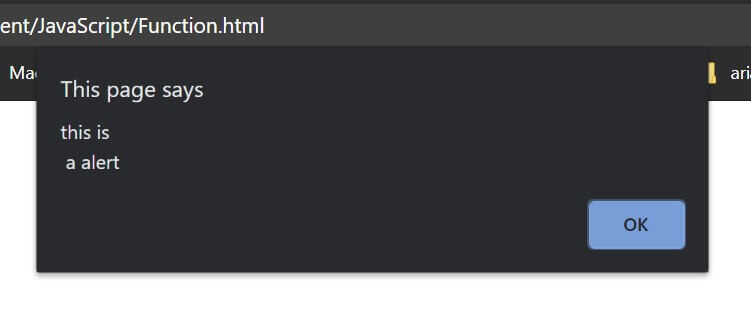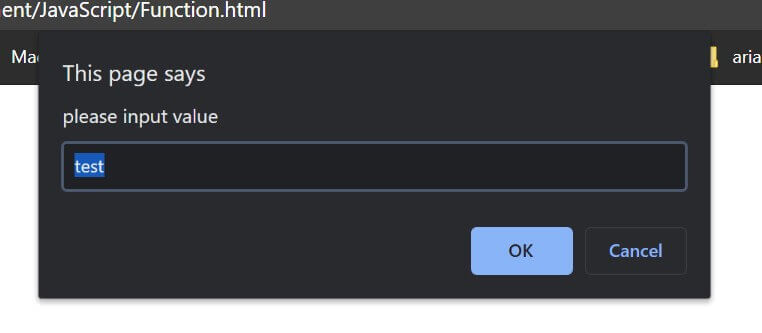# JavaScript 入门教程之四 -- Functions

JavaScript function 就是一个执行特定任务的代码块。

• 代码复用
• 通过传入不同参数得到不同结果

function 需要被调用才能执行。

### 定义

``````function name() {
//code to be executed
}``````

function name 可以包含字符、数字，下划线和 `&` 符号。

``````function myFunction() {
}

myFunction();``````

### function 参数

function 可以接收参数，在定义时需要列出来：

``````functionName(param1, param2, param3) {
// some code
}``````

``````function myFunction(name) {
}

myFunction("marco");``````

``````myFunction("marco");
myFunction("john");
myFunction("tom");``````

``````function myFunction(name, age) {
alert("my name is " + name + ", age is " + age);
}

myFunction("marco", 20);``````

JavaScript 不会检查调用 function 时的传入参数个数和定义的参数个数是否一致，如果调用时传入参数数量少于 function 定义参数个数，则缺失的参数会被赋值为 `undefined`，表示没有被赋值数据。如果传入参数数量大于 function 定义参数个数，可以使用数组：`arguments` 来访问这些传入数据，在 function 内可以被调用，形如：arguments, arguments，arguments 数组是 function 内自动定义的，按顺序存储所有的传入参数数据。

``````function test(a, b) {
console.log(arguments);
console.log(arguments);
console.log(arguments);
}
test(1, 2, 3);

//output:
//1
//2
//3``````

function 可以有一个可选的 `return` 命令，用来从 function 返回一个数据。

``````function myFunction(a, b) {
return a + b;
}

var x = myFunction(1, 2);``````

``alert("this is a alert");``

``````alert("this is\n a alert");
``````Prompt Box 用来弹出一个用户可以输入数据的提示窗口。

`prompt()` 有两个参数，第一个是窗口显示的提示信息字符串，第二个是输入框的默认字符(可选项)：

``````var a = prompt("please input value", "test");
document.write(a);``````confirm box 可以用来作为确认对话框。

``````var result = confirm("if confirm");

if (result) {
}
else {
}``````

### inline function

``````var arr = [1, 2, 3];
arr.forEach(function(el) {
console.log(el * 2);
});``````

function 直接在调用中定义，参数 el 的值通过 `forEach` method 来传入每个元素的数据。

``````    function person(name, age, color) {
this.name = name;
this.age = age;
this.favColor = color;
this.changeName = function(name) {
this.name = name;
}
}``````

### apply and call method

object 将在下一章节进行介绍：JavaScript 入门教程之五 -- Objects

``````var person = {
fullName: function () {
console.log(this.firstName + " " + this.lastName);
}
}

var person1 = {
firstName: "marco",
lastName: "nie"
}
person.fullName.apply(person1);

//output:
//marco nie``````

``````var person = {
fullName: function (city, country) {
console.log(this.firstName + " " + this.lastName + ", " + city + " " + country);
}
}

var person1 = {
firstName: "marco",
lastName: "nie"
}
person.fullName.apply(person1, ["hz", "china"]);

//output:
//marco nie, hz china``````

`call()` method 和 `apply()` 功能类似，区别是传入 function 的参数时需要分开单独传入：

``person.fullName.call(person1, "hz", "china");``

``````function test(a, b, c) {
console.log(a + b + c);
}
var arr = [1, 2, 3];
test.apply(null, arr);``````

function 的常规使用方法就介绍完毕。